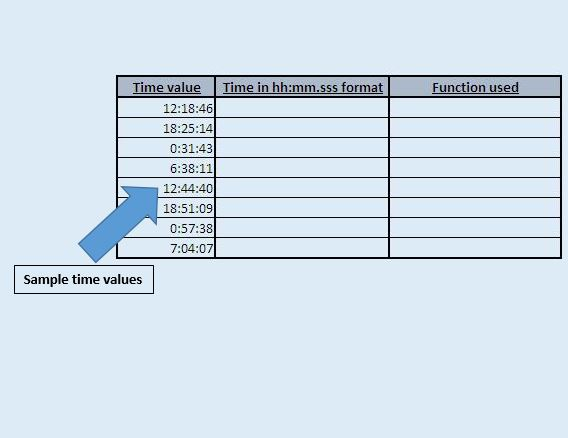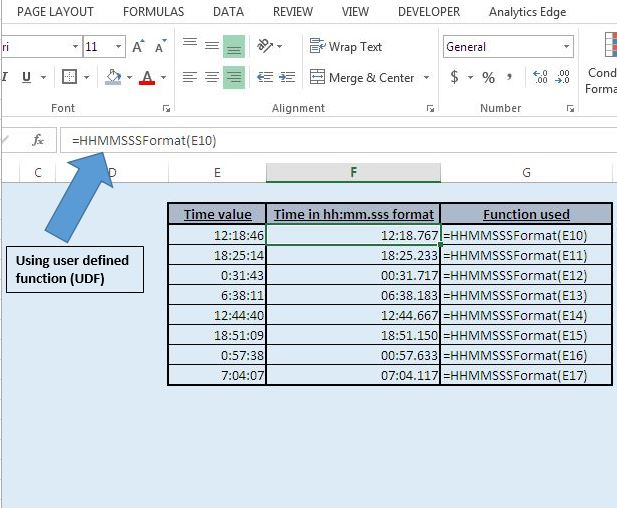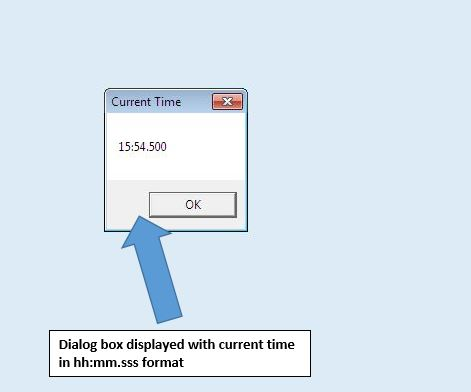# Show the time in hh:mm.sss format using VBA

In this article, we will create a macro to format the time in hh:mm.sss format.

Raw data for this example consists of certain time values in column E.In this article, we have created a user defined function (or custom function) “HHMMSSSFormat”. This function takes date type as input and returns output in string data type in hh:mm.sss format.

Logic explanation

In “HHMMSSSFormat” function to convert 60 seconds to a three digit number, we have divided seconds in the defined time value by 60 to get fraction for defined seconds and then multiplied it by thousand to get the three digit number.

“HHMMSSSFormat” function can be used by calling either directly in the Excel sheet or by using the function inside the other procedure (or macro).

The image below shows how we have used “HHMMSSSFormat” function in the Excel sheet to derive the time in hh:mm.sss format.We have also created a “GettingCurrentTimeinHHMMSSSFormat” macro which uses the “HHMMSSSFormat” function to display the current time in hh:mm.sss format in a message box. The image below shows the output when we run this macro at 3:54:30s.```
Option Explicit

Function HHMMSSSFormat(DateTime As Date) As String
'function will return string value

'Declaring integer variable
Dim SecondValue As Integer

'Extracting seconds from DateTime parameter
SecondValue = Second(DateTime)

'Converting seconds value to three digit number
SecondValue = (SecondValue / 60) * 1000

'Change the formatting of time in the required format
HHMMSSSFormat = Format(Hour(DateTime), "00") & ":" & _
Format(Minute(DateTime), "00") & "." & Format(SecondValue, "000")

End Function

Sub GettingCurrentTimeinHHMMSSSFormat()

'Declaring string variable
Dim CurrentTime As String

'Calling custom function HHMMSSSFormat
CurrentTime = HHMMSSSFormat(Now)

'Displaying message box with Ok button only
MsgBox CurrentTime, vbOKOnly, "Current Time"

End Sub

```